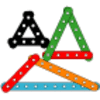#### You may also like### I'm Eight

Find a great variety of ways of asking questions which make 8.### Let's Investigate Triangles

Vincent and Tara are making triangles with the class construction set. They have a pile of strips of different lengths. How many different triangles can they make?### Noah

Noah saw 12 legs walk by into the Ark. How many creatures did he see?

# Largest Even

## Largest Even

I have a pile of nine digit cards numbered $1$ to $9$.

I take one of the cards.  It is the $3$.

Which card would you choose so you could make the largest possible two-digit even number with the two cards?

We put the cards back in the pile.  This time, I choose the $6$. Which card would you choose this time to make the largest possible two-digit even number?

Have a go at this with a partner.  One of you chooses the first digit from the set of cards.  The second person then chooses a card to make the largest possible two-digit even number. You can then swap over.

Try it several times so you are sure you have a good method. Talk about your ideas with your partner so you agree together on a 'best' method.

How would your strategy change if you had to make the largest two-digit odd number?

If you don't have a partner to work with, you could use the interactivity below.  The computer selects one digit at random.   You must then choose a digit to make the largest possible two-digit even number or largest possible two-digit odd number.

Enter the biggest two-digit number you can think of that uses the digit:

### Why do this problem?

The idea of this problem is to encourage children to work together in order to develop a method of finding a solution which will always work, and perhaps one that is the most efficient or quickest.  This challenge will also help to reinforce understanding of odd and even numbers.

### Possible approach

You could introduce the challenge by inviting one child to choose a card from the digit cards $1$ to $9$, perhaps without looking so it is a random choice.  You could then ask another child to choose a card, again without looking.  What is the largest two-digit even number that can be made from the two cards?  You could try this a few times so that the children have a chance to explain when it is impossible to make an even number.

Then tweak the task.  This time, the second person to choose can decide which digit they would like in order to make the largest possible two-digit number.  You could suggest that in fact a pair of children chooses the card so that they have a chance to talk to each other about the best choice.  You could invite others to comment on their choice.  (You could use the interactivity at this point instead.)

Ask pairs to have a go at the challenge for themselves with a set of digit cards per pair.  Explain that you'd like them to come up with a method that will always work so they can identify the best card to choose as quickly as possible.

After a suitable length of time, bring the whole group together and share their strategies.  You could test out a few of them and perhaps a consensus can be reached about the 'best' method to use.  (This may not be possible but having the discussion is valuable.  In this case 'best' might be quickest but perhaps the children may want to clarify that for themselves.)

How would they change their method if the task was to make the highest two-digit odd  number?

### Key questions

What do you know about even numbers?
What do you know about the units digit of even numbers?
Where could the digit on that first card go - in the units or tens?  Why?
How can we make a large number?
How do we know that's the biggest even number we can make?

### Possible extension

Challenge children to extend the strategy for three-digit numbers.  They could pick two numbers out of the pack of digit cards at random, then try to make the largest possible three-digit even number by selecting one of the remaining cards as well.  Another possibility would be to aim for multiples of $5$ rather than even numbers.  Some learners might want to investigate what happens if $0$ is included as well.

### Possible support

Some children might benefit from having equipment to help them check whether a number is even, for example multilink cubes or counters that can be put in pairs.